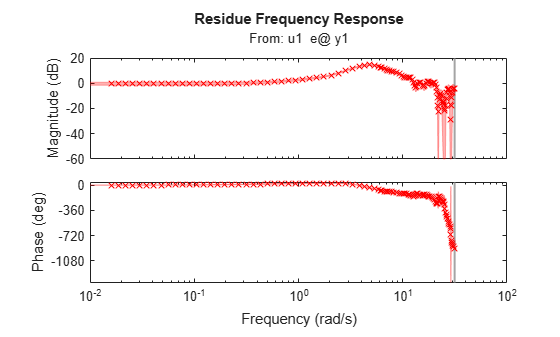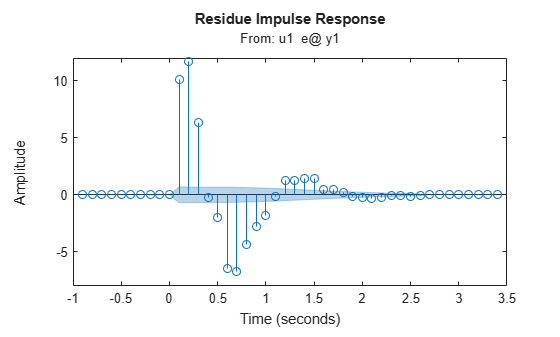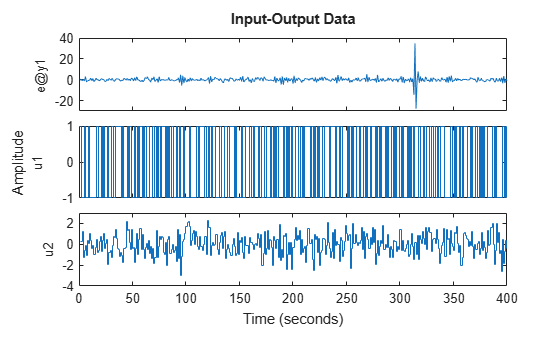Documentation

resid

Compute and test residuals

Description

example

resid(Data,sys) computes the 1-step-ahead prediction errors (residuals) for an identified model, sys, and plots residual-input dynamics as one of the following, depending on the data inData:

• For time-domain data, resid plots the autocorrelation of the residuals and the cross-correlation of the residuals with the input signals. The correlations are generated for lags -25 to 25. To specify a different maximum lag value, use residOptions. The 99% confidence region marking statistically insignificant correlations displays as a shaded region around the X-axis.

• For frequency-domain data, resid plots a bode plot of the frequency response from the input signals to the residuals. The 99% confidence region marking statistically insignificant response is shown as a region around the X-axis.

To change display options, right-click the plot to access the context menu. For more details about the menu, see Tips.

example

resid(Data,sys,Linespec) sets the line style, marker symbol, and color.

example

resid(Data,sys1,...,sysn) computes and plots the residual of multiple identified models sys1,...,sysn.

example

resid(Data,sys1,Linespec1,...,sysn,Linespecn) sets the line style, marker symbol, and color for each system.

example

resid(___,Options) specifies additional residual calculation options. Use Options with any of the previous syntaxes.

example

resid(___,Type) specifies the plot type. Use Type with any of the previous syntaxes.

example

[E,R] = resid(Data,sys) returns the calculated residuals, E, and residual correlations, R. No plot is generated.

Examples

collapse all

data = z1;

Estimate an ARX model.

sys = arx(data,[1 1 0]);

Plot the autocorrelation of the residuals and cross-correlation between the residuals and the inputs.

resid(data,sys)The correlations are calculated until the default maximum lag, 25. The 99% confidence region marking statistically insignificant correlations displays as a shaded region around the X-axis.

Convert data to frequency domain.

data2 = fft(data);

Compute the residuals for identified model, sys, and the frequency-domain data. Plot the residual response using red crosses.

resid(data2,sys,'rx')For frequency-domain data, resid plots the Bode plot showing frequency response from the input to the residuals.

Estimate an ARX model.

sys1 = arx(z1,[1 1 0]);

Estimate a transfer function model.

sys2 = tfest(z1,2);

Plot the correlations of the residuals.

resid(z1,sys1,'b',sys2,'r')The cross-correlation between residuals of sys2 and the inputs lie in the 99% confidence band for all lags.

Estimate an ARX model.

sys = arx(z1,[1 1 0]);

Specify the maximum lag for residual correlation calculations.

opt = residOptions('MaxLag',35);

Plot the impulse response from the input to the residuals.

resid(z1,sys,opt,'ir')The data is a two-input, single-output dataset.

Estimate an ARX model.

sys = tfest(z7,2);

Calculate the residuals and their autocorrelations and cross-correlations with inputs.

[E,R] = resid(z7,sys);

R is a 26-by-3-by-3 matrix of correlations. For example,

• R(:,1,1) is the autocorrelation of the residuals until lag 25.

• R(:,1,2) is the cross-correlation of the residuals with the first input,until lag 25.

E is an iddata object with the residuals as output data and the inputs of validation data (z7) as input data. You can use E to identify error models and analyze the error dynamics.

Plot the error.

plot(E)Estimate impulse response between inputs and residuals. Plot them with a 3 standard deviation confidence region.

I = impulseest(E);
showConfidence(impulseplot(I,20),3)Input Arguments

collapse all

Validation input-output data, specified as an iddata object. Data can have multiple input-output channels. When sys is linear, Data is time-domain or frequency-domain. When sys is nonlinear, Data is time-domain.

System used for computing residuals, specified as an identified linear or nonlinear model.

Example: idpoly

Line style, marker symbol, and color, specified as a character vector. For more information, see plot in the MATLAB® documentation. When Type is specified as 'corr', only the line style is used.

Example: 'Linespec','kx'

Residual analysis options, specified as an residOptions option set.

Plot type, specified as one of the following values:

• 'corr' — Plots the autocorrelation of the residuals, e, and the cross-correlation of the residuals with the input signals, u. The correlations are generated for lags -25 to 25. Use residOptions to specify a different maximum lag value. The 99% confidence region marking statistically insignificant correlations is also shown as a shaded region around the X-axis. The computation of the confidence region is done assuming e to be white and independent of u.

'corr' is default for time-domain data. This plot type is not available for frequency-domain data.

• 'ir' — Plots the impulse response up to lag 25 of a system from the input to the residuals. The impulseest command first estimates the impulse response model with e as output data and u as inputs. Then impulseest calculates the impulse response of the estimated model. The 99% confidence region marking statistically insignificant response displays as a shaded region. A low magnitude indicates a reliable model.

This plot type is not available for frequency-domain data.

• 'fr' — The frequency response from the input to the residuals (based on a high-order FIR model) is shown as a Bode plot. The 99% confidence region marking statistically insignificant response displays as a shaded region. A low magnitude in the frequency range of interest indicates a reliable model.

'fr' is default for frequency-domain data.

Output Arguments

collapse all

Model residuals, returned as an iddata object. The residuals are stored in E.OutputData, and the inputs are stored in E.InputData. Use E to build models that describe the dynamics from the inputs to the residuals. The dynamics are negligible if sys is a reliable identified model.

Correlations of the residuals, returned as one of the following:

• Matrix of doubles — For time-domain-data

R is a matrix of size M+1-by-(ny+nu)-by-(ny+nu). Where, M is the maximum lag specified in Options, ny is the number of outputs, and nu is the number of inputs. The default value of M is 25.

At each lag k (k = 0:M), R(k,i,j) is the expectation value, <Z(t,i).Z(t+k-1,j)>. Here, Z = [E.OutputData,E.InputData].

For example, for a two-output, single-input model, Z = [e1,e2,u1]. Where, e1 is the residual of the first output, e2 is the residual of the second output, and u1 is the input. R is a 26-by-3-by-3 matrix, where:

• R(5,1,2) = <e1(t).e2(t+4)> is the cross-correlation at lag 4 between e1 and e2.

• R(5,1,3) = <e1(t).u1(t+4)> is the cross-correlation at lag 4 between e1 and u1.

• R(5,1,1), R(5,2,2), R(5,3,3) are the autocorrelations at lag 4 for e1, e2, and u1, respectively.

• [] — For frequency-domain data

Tips

• Right-clicking the plot opens the context menu, where you can access the following options:

• Systems — Select systems to view the residual correlation or response plots. By default, all systems are plotted.

• Show Confidence Region — View the 99% confidence region marking statistically insignificant correlations. Applicable only for the correlation plots.

• Data Experiment — For multi-experiment data only. Toggle between data from different experiments.

• Characteristics — View data characteristics. Not applicable for correlation plots.

• Peak Response — View peak response of the data.

• Confidence Region — View the 99% confidence region marking statistically insignificant response.

• Show — Applicable only for frequency-response plots.

• Magnitude — View magnitude of frequency response.

• Phase — View phase of frequency response.

• I/O Grouping — For datasets containing more than one input or output channel. Select grouping of input and output channels on the plot. Not applicable for correlation plots.

• None — Plot input-output channels in their own separate axes.

• All — Group all input channels together and all output channels together.

• I/O Selector — For datasets containing more than one input or output channel. Select a subset of the input and output channels to plot. By default, all output channels are plotted.

• Grid — Add grids to the plot.

• Normalize — Normalize the y-scale of all data in the plot. Not applicable for frequency-response data.

• Full View — Return to full view. By default, the plot is scaled to full view.

• Initial Condition — Specify handling of initial conditions.

Specify as one of the following:

• Estimate — Treat the initial conditions as estimation parameters.

• Zero — Set all initial conditions to zero.

• Absorb delays and estimate — Absorb nonzero delays into the model coefficients and treat the initial conditions as estimation parameters. Use this option for discrete-time models only.

• Properties — Open the Property Editor dialog box to customize plot attributes.

 Ljung, L. System Identification: Theory for the User. Upper Saddle River, NJ: Prentice-Hall PTR, 1999, Section 16.6.

System Identification Toolbox DocumentationGet trial now